# De L'Hospital’s rule

Let at x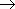a for functions f ( x ) and g ( x ), differentiable in some neighborhood of the point a , the conditions are executed: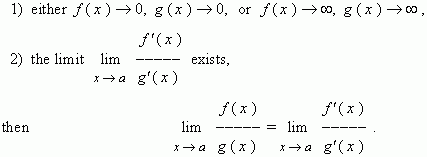This theorem is called de L'Hospital’s rule . It allows to calculate limits of ratios of functions, when both a numerator and a denominator approach either zero, or infinity. As mathematicians say, de L'Hospital’s rule permits to get rid of indeterminacies of types 0 / 0 and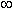/.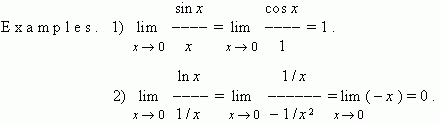At indeterminacies of other types:,× 0 , 0 0 ,0 ,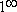it is necessary to do some identical transformations to reduce them to one of these two indeterminacies: either  0 / 0 , or/. After this it is possible to use de L'Hospital’s rule. Show some of possible transformations of the above mentioned indeterminacies.

 1)–: let f ( x ), g ( x ), then this indeterminacy is reduced to the type 0 / 0 by the following  transformation: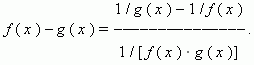2)× 0 : let f ( x ), g ( x )0 , then this indeterminacy is reduced to the types 0 / 0  or/by the following  transformations: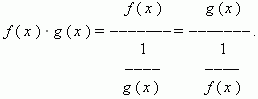3) the rest of the indeterminacies are reduced to the first ones by the logarithmic transformation: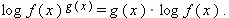If after using of de L'Hospital’s rule the indeterminacies of the types 0 / 0 or/remain, it is necessary to repeat it. The multifold use of de L'Hospital’s rule can give the required result. The de L'Hospital’s rule is also applicable, if x.